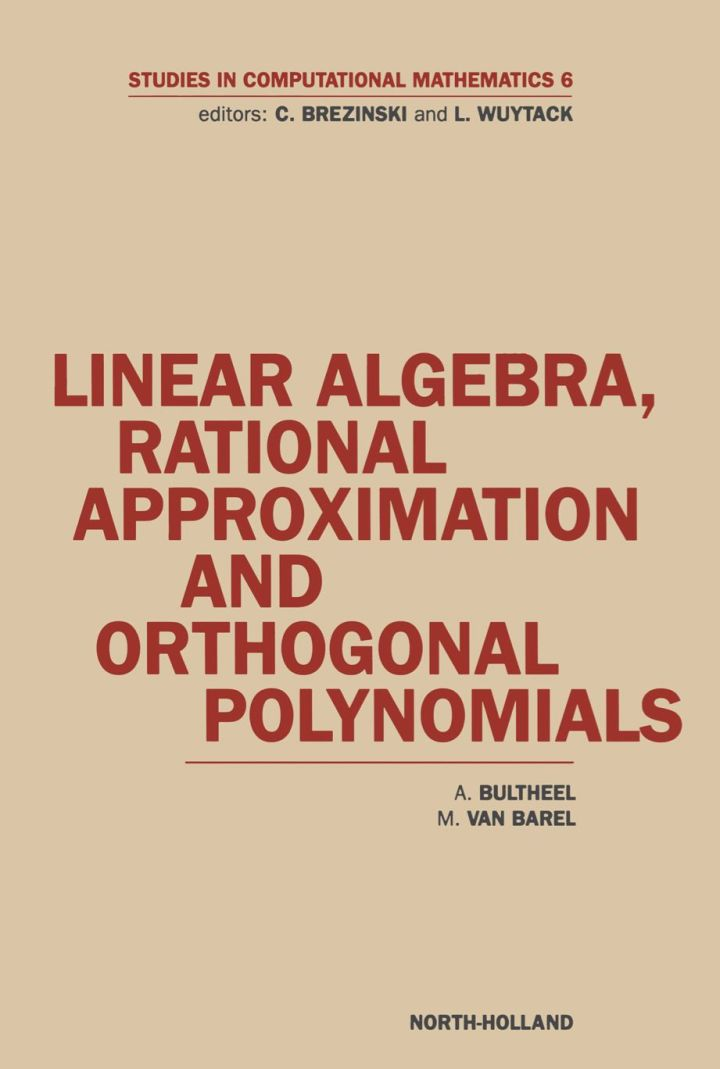# Linear Algebra, Rational Approximation and Orthogonal Polynomials

## Linear Algebra, Rational Approximation and Orthogonal Polynomials

By Bultheel, A.; Van Barel, M.

### Rent or Buy eTextbook

Expires on Apr 18th, 2022
\$255

Publisher List Price: \$279.99
Savings: \$24.99

Evolving from an elementary discussion, this book develops the Euclidean algorithm to a very powerful tool to deal with general continued fractions, non-normal Padé tables, look-ahead algorithms for Hankel and Toeplitz matrices, and for Krylov subspace methods. It introduces the basics of fast algorithms for structured problems and shows how they deal with singular situations.

Links are made with more applied subjects such as linear system theory and signal processing, and with more advanced topics and recent results such as general bi-orthogonal polynomials, minimal Padé approximation, polynomial root location problems in the complex plane, very general rational interpolation problems, and the lifting scheme for wavelet transform computation. The text serves as a supplement to existing books on structured linear algebra problems, rational approximation and orthogonal polynomials.

Features of this book:

• provides a unifying approach to linear algebra, rational approximation and orthogonal polynomials

• requires an elementary knowledge of calculus and linear algebra yet introduces advanced topics.

The book will be of interest to applied mathematicians and engineers and to students and researchers.

Subject: Mathematics & Statistics -> Calculus -> Introductory Linear AlgebraPublisher: Elsevier S & T 11/1997
Imprint: North Holland
Language: English
Length: 445 pages

ISBN 10: 0444828729
ISBN 13: 9780444828729
Print ISBN: 9780444828729

Live Chats